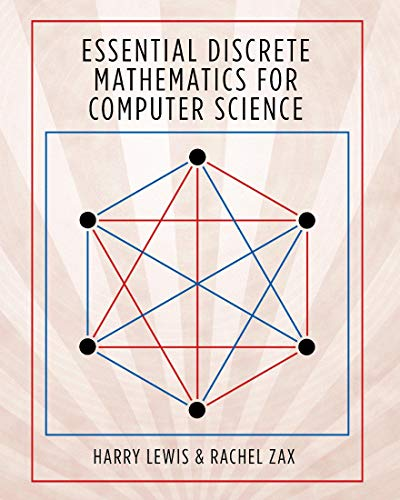Please Enter ISBN, Title or Author’s Nameand more...

# Essential Discrete Mathematics for Computer ScienceAuthors: Harry Lewis,Rachel Zax
ISBN:0691179298
ISBN-13: 9780691179292
List Price: \$75.00 (up to 15% savings)
Prices shown are the lowest from
the top textbook retailers.

## View all Prices by RetailereTextbook \$75.00## Details about Essential Discrete Mathematics for Computer Science:

A more intuitive approach to the mathematical foundation of computer science

Discrete mathematics is the basis of much of computer science, from algorithms and automata theory to combinatorics and graph theory. This textbook covers the discrete mathematics that every computer science student needs to learn. Guiding students quickly through thirty-one short chapters that discuss one major topic each, this flexible book can be tailored to fit the syllabi for a variety of courses.

Proven in the classroom, Essential Discrete Mathematics for Computer Science aims to teach mathematical reasoning as well as concepts and skills by stressing the art of proof. It is fully illustrated in color, and each chapter includes a concise summary as well as a set of exercises. The text requires only precalculus, and where calculus is needed, a quick summary of the basic facts is provided.

Essential Discrete Mathematics for Computer Science is the ideal introductory textbook for standard undergraduate courses, and is also suitable for high school courses, distance education for adult learners, and self-study.

• The essential introduction to discrete mathematics
• Features thirty-one short chapters, each suitable for a single class lesson
• Includes more than 300 exercises
• Almost every formula and theorem proved in full
• Breadth of content makes the book adaptable to a variety of courses
• Each chapter includes a concise summary
• Solutions manual available to instructors

Need Machine Theory tutors? Start your search below:
Need Machine Theory course notes? Start your search below: